# Energy Converter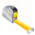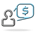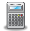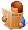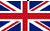Converter Currencies Calculator Articles

 Other units Area Energy Flowrate Force Length Mass Power Pressure Velocity Volume Temperature Time ______________ AcresAresBars Barrels per day Baryes BTUs BTUs per hour BTUs per minute BTUs per second Centimeters (cm) cm/sec Chains Cooking measures Crinals Cubic centimeters (cc) cc/sec cc-atmosphere Cubic decimeters Cubic feet Cubic feet per minute Cubic feet per second Cubic inches Cubic inches per minute Cubic inches per second Cubic meters Cubic meters per hour Cubic yards Cubic yards per minute Decimeters (dm) Drams DynesErgs per second Fluid drachmas Fluid ounces (fl.oz.) Feet (ft) Feet per hour Feet per minute Feet per second ft-lbf ft-lbf/hour ft-lbf/min ft-lbf/sec Furlongs Gallons Gallons per day Gallons per minute Gallons per second Gigawatts (GW) Gills Grains Grams (g) Gram-calories (g-cal) Hectares Hectometers Hundredweights (cwt) Inches (in) Inches H2O Inches Hg Inch-pound-forces (in-lbf) IST calories Joules (J) Joules per second (J/sec) Kilocalories (kcal) Kilograms (kg) kg-meters/sq. sec Kilogram-calories Kilogram-forces (kgf) Kgf/sq. cm Kgf/sq. meter Kilometers (km) Kilometers per hour (km/h) KiloPascal(kPa) Kilowatts (kW) Kilowatts per hour (kWh) Kips Knots Liters (l) Liters per minute Liters per second Long tons (imperial tons) MegaPascal(mPa) Megawatts (MW) Meters (m) Meters per minute Meters per second Metric horsepower Miles Miles per hour (mph) Miles per minute Milligrams (mg) Milliliters (ml) Millimeters (mm) Miner inches Newton (N) Ounces (oz) Ounce-force Pascal (Pa) Pints Pounds (lb) Pounds/sq. foot Pounds/sq. inch Poundals Poundals/sq. foot Poundals/sq. inch Pound-CHU Pound-force (lbf) Quarts Quarters Rods Shoe size Short tons/sq. footSlugs Square centimeters Square decimeters Square feet Square kilometers Square meters Square miles Square millimeters Square yards Stones Thermocalories TonsTons (refrigeration) Tonnes (metric tons) Torrs U.S. gallons U.S. horsepower per hour U.S. tons Watts Watts per hour Yards ________________ Ancient Greek: area Ancient Greek: currency Ancient Greek: length Ancient Greek: mass Ancient Greek: volume Ancient Roman: area Ancient Roman: length Ancient Roman: mass/coin Ancient Roman: volume Apothecary: imperial UK Apothecary: US/UK Apothecary: weightLeague units ________________ Calculator Contact Main website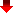In the energy sector, converting energy units can provide an accurate estimate of energy consumption, which is crucial for energy efficiency and cost analysis. In engineering, converting energy units helps to design systems that use energy efficiently and avoid waste. Converting energy units can also help to determine the energy required to operate a system, which is essential for designing energy-efficient systems.

In research, converting energy units helps to compare and analyze data from different sources, which is crucial for advancing knowledge in various fields. For instance, it can help researchers to compare energy consumption data from different countries, which is important for understanding energy consumption patterns and developing energy-saving policies.

Furthermore, converting energy units helps to ensure that data is communicated accurately between different stakeholders, which is crucial for making informed decisions. For example, converting energy units can help to ensure that energy consumption data is accurately reported to stakeholders, which is important for transparency and accountability.

The conversion of energy units helps to ensure that data is consistent, which is important for reliable and accurate decision-making.

MetricToImperial.com